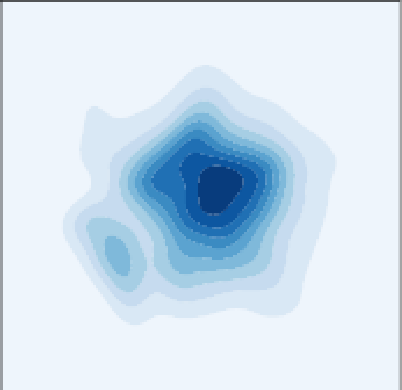2 May 2019

# InfoVAE: Balancing Learning and Inference in Variational Autoencoders

Zhao et al, AAAI 2019, [link]
tags: representation learning - aaai - 2017Two known shortcomings of VAEs are that (i) The variational bound (ELBO) can lead to poor approximation of the true likelihood and inaccurate models and (ii) the model can ignore the learned latent representation when the decoder is too powerful. In this work, the author propose to tackle these problems by adding an explicit mutual information term to the standard VAE objective.
• Pros (+): Well justified, fast implementation trick, semi-supervised setting.
• Cons (-): requires explicit knowledge of the sensitive attribute.

### InfoVAE

Intuitively, the InfoVAE objective can be seen as a variant of the standard VAE objective with two main modifications (i) an additional term that strives to maximize the mutual information between the input $x$ and latent $z$, to force the model to make use of the latent representation, and (ii) a weighting between the reconstruction and latent loss term to better balance their contribution (similar to $\beta$-VAE )

The first two terms correspond to a weighted variant of the ELBO, while the last term adds a constraint on the latent codes distribution. Since estimating $q_{\phi}(z)$ would require marginalizing $q_{\phi}(z | x)$ over all $x$, it is instead approximated by sampling (first $x$ then $z$, from the encoder distribution). Furthermore, the authors show that the objective is still valid when replacing these last terms by any other hard divergence. In particular, this makes it a generalization of Adversarial Auto-encoders (AAE) , which uses the Jensen Divergence (approximated by an adversary).

### Experiments

The authors experiment with three different divergences: Jensen ($\simeq$ AAE), Stein Variational Gradient and Maximum-Mean Discrepancy. Results seem to indicate that InfoVAE leads to more principled latent representations, and better balance between reconstruction and latent space usage. As such, reconstructions might not look as crisp than ones from vanilla VAE but generated samples are of better quality (better generalization, also can be seen in semi-supervised task that make use of the representation)

### References

•  $\beta$-VAE: Learning basic visual concepts with a constrained variational framework, Higgins et al, ICLR 2017
•  Adversarial Autoencoders, Mahkzani et al, ICLR 2016